Next: Cook's Theorem Up: Subset-Sum Problems and NP-Completeness Previous: Converting (SP) to (SSP)   Contents

## Converting (SSP) to (SP)

Suppose we have a subset-sum problem with 50, all between 1 and, with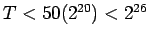. Solving the SSP may be crudely broken into two steps:
1. Decide whichare in the subset.
2. Verify that the sum of the chosenis.
Our (SP) will also carry out these steps. The first is simple: we will have logic variableswith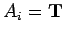corresponding tobeing in the subset. To carry out the second step, we need to construct a set of logic formulas which acts as an adding machine'' to check the total.

We will represent numbers in base 2. Since all relevant numbers are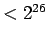, numbers may be represented by 26 logical variables. For each, we will have variables. These will representif, 0 if. To do this, we need formulas which show how the value of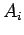determines the value of all the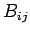:with the simple formula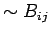if the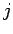th digit ofis 0.

Next, we need, for, variables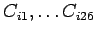which represent the total of the first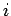of the numbers given by the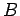-variables. Formulas are needed which show the-variables determining the-variables.

We begin with a set of formulaswhich have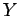get value T if and only if an odd number ofhave value T.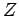gets value T if and only if 2 or 3 ofhave value T:Ifrepresent three one-digit numbers (0 or 1), the formulashave the effect thatis the number in the column with the three numbers, whileshows whether there is a number carried into the next column.

We will use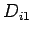,...,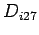,, to keep track of numbers being carried. Since there are no numbers carried in the rightmost column, we have the formulas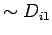. The formulas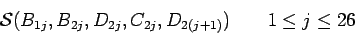have the effect of makingrepresent the sum of the numbersand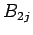. To have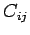represent the sum ofand, we useThese logic formulas together have the effect that thedetermine the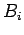, which determinethroughsuccessively. This last group of variables corresponds to the base-2 representation of the sum of thewhich are in the set we have chosen. Finally, we add the formulasor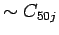depending on whether theth digit ofis 1 or 0. As planned, a solution to this satisfiability problem gives a solution to the subset-sum problem (look at whichhave value T), which implies that a method of solving (SP) can be used to solve (SSP).

The (SP) we have constructed is rather large, involving approximately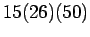formulas. However, the rate of growth if we had morewith a larger upper limit is not too bad.Next: Cook's Theorem Up: Subset-Sum Problems and NP-Completeness Previous: Converting (SP) to (SSP)   Contents
Translated from LaTeX by Scott Sutherland
2002-12-14